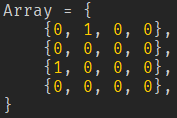# Making a 2D array 'Bigger'

I’d like to find out how I would go about turning an array like this:into an array like this:Well, you are just doubling the entires in each direction. So you just need to do that in code.

``````local function stretchNestedArray(array)
local out = {}
for _, row in pairs(array) do
local extendedRow = {}
for _, entry in pairs(row) do
table.insert(extendedRow, entry)
table.insert(extendedRow, entry)
end
table.insert(out, extendedRow)
table.insert(out, extendedRow)
end
return out
end
``````
1 Like

And because I was bored have a recursive version too. The advantage to the recursive one is that you can use any amount of nesting (well, to a very generous limit)

``````local function recursiveStretch(array, stretchFactor)
stretchFactor = stretchFactor or 2 --default to 2

local out = {}
for _, val in pairs(array) do
if type(val) == "table" then
val = recursiveStretch(val, stretchFactor)
end

for i=1, stretchFactor do
table.insert(out, val)
end
end
return out
end
``````
1 Like
``````local function create2DArray(rows, columns)
local t = {}
for i = 1, rows do
local row = table.create(columns, 0)
table.insert(t, row)
end
return t
end

local t = create2DArray(3, 3)
print(t)
--[[
{
{0, 0, 0},
{0, 0, 0},
{0, 0, 0}
}
]]
``````